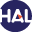# Volume 14 (2017)

Latest addition : 17 October 2017.

• ### Robust MUSCL Schemes for Ten-Moment Gaussian Closure Equations with Source Terms

17 October 2017,

Abstract:

In this article, we present positivity preserving second-order numerical schemes to approximate Ten-Moment Gaussian closure equations with source terms. The challenge here is to preserve the positivity of the density and the symmetric pressure tensor. We propose MUSCL type numerical schemes to overcome these difficulties. The principal components of the proposed schemes are a Strang splitting of the source terms, positivity preserving first order scheme and suitable linear reconstruction process which ensures the positivity of the reconstructed variables. To achieve positivity of reconstructed variables, we impose the additional restrictions on the slopes of the linear reconstructions. Additionally, the source is discretized using both explicit and implicit methods. In the case of explicit source discretization, we derive the appropriate condition on the time step for discretization to be positivity preserving. Implicit discretization of the source terms is shown to be unconditionally positivity preserving. Numerical examples are presented to demonstrate the superior robustness and stability of the proposed numerical schemes.

Key words : Ten-Moment equations, Finite Volume Methods, MUSCL Scheme, Positivity preserving schemes.

Paper presented by: Thierry GallouëtVoir sur HAL : https://hal.archives-ouvertes.fr/hal-01619021